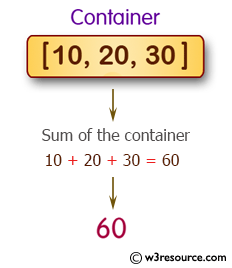﻿ Python: Calculate the sum over a container - w3resource# Python: Calculate the sum over a container

## Python Basic: Exercise-82 with Solution

Write a Python program to calculate the sum of all items of a container (tuple, list, set, dictionary).

Some objects contain references to other objects; these are called containers. Generally, containers provide a way to access the contained objects and to iterate over them. Examples of containers are lists, sets, tuples and dictionaries.

Pictorial Presentation:Sample Solution:

Python Code:

``````s = sum([10,20,30])
print("\nSum of the container: ", s)
print()
```
```

Sample Output:

```Sum of the container:  60
```

## Visualize Python code execution:

The following tool visualize what the computer is doing step-by-step as it executes the said program:

list container:

Python Code:

``````nums = [10,20,30]
print("Original container:")
print(nums)
print(type(nums))
print("Sum of all items of the said container:", sum(nums))
```
```

Sample Output:

```Original container:
[10, 20, 30]
<class 'list'>
Sum of all items of the said container: 60
```

dictionary container:

Python Code:

``````def dict_sum(nums):
num_sum = 0
for i in nums:
num_sum = num_sum + nums[i]
return num_sum
nums = {'a': 100, 'b':200, 'c':300, 'd':120}
print("Original container:")
print(nums)
print(type(nums))
print("Sum of all items of the said container:", dict_sum(nums))
```
```

Sample Output:

```Original container:
{'a': 100, 'b': 200, 'c': 300, 'd': 120}
<class 'dict'>
Sum of all items of the said container: 720
```

set container:

Python Code:

``````nums = {7, 4, 9, 1, 3, 2}
print("The original container")
print(nums)
print(type(nums))
sum_tuple = sum(nums)
print("Sum of all items of the said container:", str(sum_tuple))
```
```

Sample Output:

```The original container
{1, 2, 3, 4, 7, 9}
<class 'set'>
Sum of all items of the said container: 26
```

tuple container:

Python Code:

``````nums = (7, 4, 9, 1, 3, 2)
print("The original container")
print(nums)
print(type(nums))
sum_tuple = sum(nums)
print("Sum of all items of the said container:", str(sum_tuple))
```
```

Sample Output:

```The original container
(7, 4, 9, 1, 3, 2)
<class 'tuple'>
Sum of all items of the said container: 26
```

Python Code Editor:

Have another way to solve this solution? Contribute your code (and comments) through Disqus.

What is the difficulty level of this exercise?

Test your Python skills with w3resource's quiz

﻿

## Python: Tips of the Day

Try-catch-else construct:

```try:
foo()
except Exception:
print("Exception occured")
else:
print("Exception didnt occur")
finally:
print("Always gets here")
```